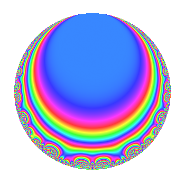# Properties

 Label 600.2.b.cLevel 600 Weight 2 Character orbit 600.b Analytic conductor 4.791 Analytic rank 0 Dimension 4 CM disc. -15 Inner twists 8

# Learn more about

## Newspace parameters

 Level: $$N$$ = $$600 = 2^{3} \cdot 3 \cdot 5^{2}$$ Weight: $$k$$ = $$2$$ Character orbit: $$[\chi]$$ = 600.b (of order $$2$$ and degree $$1$$)

## Newform invariants

 Self dual: No Analytic conductor: $$4.79102412128$$ Analytic rank: $$0$$ Dimension: $$4$$ Coefficient field: $$\Q(\sqrt{-3}, \sqrt{5})$$ Coefficient ring: $$\Z[a_1, a_2, a_3]$$ Coefficient ring index: $$2^{2}$$ Sato-Tate group: $\mathrm{U}(1)[D_{2}]$

## $q$-expansion

Coefficients of the $$q$$-expansion are expressed in terms of a basis $$1,\beta_1,\beta_2,\beta_3$$ for the coefficient ring described below. We also show the integral $$q$$-expansion of the trace form.

 $$f(q)$$ $$=$$ $$q$$ $$-\beta_{2} q^{2}$$ $$+ ( \beta_{1} - \beta_{2} ) q^{3}$$ $$+ \beta_{3} q^{4}$$ $$+ ( -2 + \beta_{3} ) q^{6}$$ $$+ ( 2 \beta_{1} - \beta_{2} ) q^{8}$$ $$-3 q^{9}$$ $$+O(q^{10})$$ $$q$$ $$-\beta_{2} q^{2}$$ $$+ ( \beta_{1} - \beta_{2} ) q^{3}$$ $$+ \beta_{3} q^{4}$$ $$+ ( -2 + \beta_{3} ) q^{6}$$ $$+ ( 2 \beta_{1} - \beta_{2} ) q^{8}$$ $$-3 q^{9}$$ $$+ ( 2 \beta_{1} + \beta_{2} ) q^{12}$$ $$+ ( -4 + \beta_{3} ) q^{16}$$ $$+ ( 4 \beta_{1} - 4 \beta_{2} ) q^{17}$$ $$+ 3 \beta_{2} q^{18}$$ $$-4 q^{19}$$ $$+ ( -4 \beta_{1} - 4 \beta_{2} ) q^{23}$$ $$+ ( -4 - \beta_{3} ) q^{24}$$ $$+ ( -3 \beta_{1} + 3 \beta_{2} ) q^{27}$$ $$+ ( 2 - 4 \beta_{3} ) q^{31}$$ $$+ ( 2 \beta_{1} + 3 \beta_{2} ) q^{32}$$ $$+ ( -8 + 4 \beta_{3} ) q^{34}$$ $$-3 \beta_{3} q^{36}$$ $$+ 4 \beta_{2} q^{38}$$ $$+ ( 8 + 4 \beta_{3} ) q^{46}$$ $$+ ( -4 \beta_{1} - 4 \beta_{2} ) q^{47}$$ $$+ ( -2 \beta_{1} + 5 \beta_{2} ) q^{48}$$ $$+ 7 q^{49}$$ $$-12 q^{51}$$ $$+ ( -2 \beta_{1} - 2 \beta_{2} ) q^{53}$$ $$+ ( 6 - 3 \beta_{3} ) q^{54}$$ $$+ ( -4 \beta_{1} + 4 \beta_{2} ) q^{57}$$ $$+ ( -4 + 8 \beta_{3} ) q^{61}$$ $$+ ( -8 \beta_{1} + 2 \beta_{2} ) q^{62}$$ $$+ ( -4 - 3 \beta_{3} ) q^{64}$$ $$+ ( 8 \beta_{1} + 4 \beta_{2} ) q^{68}$$ $$+ ( -4 + 8 \beta_{3} ) q^{69}$$ $$+ ( -6 \beta_{1} + 3 \beta_{2} ) q^{72}$$ $$-4 \beta_{3} q^{76}$$ $$+ ( 2 - 4 \beta_{3} ) q^{79}$$ $$+ 9 q^{81}$$ $$+ ( -2 \beta_{1} + 2 \beta_{2} ) q^{83}$$ $$+ ( 8 \beta_{1} - 12 \beta_{2} ) q^{92}$$ $$+ ( -6 \beta_{1} - 6 \beta_{2} ) q^{93}$$ $$+ ( 8 + 4 \beta_{3} ) q^{94}$$ $$+ ( 4 - 5 \beta_{3} ) q^{96}$$ $$-7 \beta_{2} q^{98}$$ $$+O(q^{100})$$ $$\operatorname{Tr}(f)(q)$$ $$=$$ $$4q$$ $$\mathstrut +\mathstrut 2q^{4}$$ $$\mathstrut -\mathstrut 6q^{6}$$ $$\mathstrut -\mathstrut 12q^{9}$$ $$\mathstrut +\mathstrut O(q^{10})$$ $$4q$$ $$\mathstrut +\mathstrut 2q^{4}$$ $$\mathstrut -\mathstrut 6q^{6}$$ $$\mathstrut -\mathstrut 12q^{9}$$ $$\mathstrut -\mathstrut 14q^{16}$$ $$\mathstrut -\mathstrut 16q^{19}$$ $$\mathstrut -\mathstrut 18q^{24}$$ $$\mathstrut -\mathstrut 24q^{34}$$ $$\mathstrut -\mathstrut 6q^{36}$$ $$\mathstrut +\mathstrut 40q^{46}$$ $$\mathstrut +\mathstrut 28q^{49}$$ $$\mathstrut -\mathstrut 48q^{51}$$ $$\mathstrut +\mathstrut 18q^{54}$$ $$\mathstrut -\mathstrut 22q^{64}$$ $$\mathstrut -\mathstrut 8q^{76}$$ $$\mathstrut +\mathstrut 36q^{81}$$ $$\mathstrut +\mathstrut 40q^{94}$$ $$\mathstrut +\mathstrut 6q^{96}$$ $$\mathstrut +\mathstrut O(q^{100})$$

Basis of coefficient ring in terms of a root $$\nu$$ of $$x^{4}\mathstrut -\mathstrut$$ $$x^{3}\mathstrut +\mathstrut$$ $$2$$ $$x^{2}\mathstrut +\mathstrut$$ $$x\mathstrut +\mathstrut$$ $$1$$:

 $$\beta_{0}$$ $$=$$ $$1$$ $$\beta_{1}$$ $$=$$ $$\nu^{2} - \nu + 1$$ $$\beta_{2}$$ $$=$$ $$\nu^{3} - \nu^{2} + \nu + 1$$ $$\beta_{3}$$ $$=$$ $$\nu^{3} - \nu^{2} + 3 \nu + 1$$
 $$1$$ $$=$$ $$\beta_0$$ $$\nu$$ $$=$$ $$($$$$\beta_{3}\mathstrut -\mathstrut$$ $$\beta_{2}$$$$)/2$$ $$\nu^{2}$$ $$=$$ $$($$$$\beta_{3}\mathstrut -\mathstrut$$ $$\beta_{2}\mathstrut +\mathstrut$$ $$2$$ $$\beta_{1}\mathstrut -\mathstrut$$ $$2$$$$)/2$$ $$\nu^{3}$$ $$=$$ $$\beta_{2}\mathstrut +\mathstrut$$ $$\beta_{1}\mathstrut -\mathstrut$$ $$2$$

## Character Values

We give the values of $$\chi$$ on generators for $$\left(\mathbb{Z}/600\mathbb{Z}\right)^\times$$.

 $$n$$ $$151$$ $$301$$ $$401$$ $$577$$ $$\chi(n)$$ $$-1$$ $$-1$$ $$-1$$ $$1$$

## Embeddings

For each embedding $$\iota_m$$ of the coefficient field, the values $$\iota_m(a_n)$$ are shown below.

For more information on an embedded modular form you can click on its label.

Label $$\iota_m(\nu)$$ $$a_{2}$$ $$a_{3}$$ $$a_{4}$$ $$a_{5}$$ $$a_{6}$$ $$a_{7}$$ $$a_{8}$$ $$a_{9}$$ $$a_{10}$$
251.1
 −0.309017 + 0.535233i −0.309017 − 0.535233i 0.809017 − 1.40126i 0.809017 + 1.40126i
−1.11803 0.866025i 1.73205i 0.500000 + 1.93649i 0 −1.50000 + 1.93649i 0 1.11803 2.59808i −3.00000 0
251.2 −1.11803 + 0.866025i 1.73205i 0.500000 1.93649i 0 −1.50000 1.93649i 0 1.11803 + 2.59808i −3.00000 0
251.3 1.11803 0.866025i 1.73205i 0.500000 1.93649i 0 −1.50000 1.93649i 0 −1.11803 2.59808i −3.00000 0
251.4 1.11803 + 0.866025i 1.73205i 0.500000 + 1.93649i 0 −1.50000 + 1.93649i 0 −1.11803 + 2.59808i −3.00000 0
 $$n$$: e.g. 2-40 or 990-1000 Significant digits: Format: Complex embeddings Normalized embeddings Satake parameters Satake angles

## Inner twists

Char. orbit Parity Mult. Self Twist Proved
1.a Even 1 trivial yes
15.d Odd 1 CM by $$\Q(\sqrt{-15})$$ yes
3.b Odd 1 yes
5.b Even 1 yes
8.d Odd 1 no
24.f Even 1 no
40.e Odd 1 no
120.m Even 1 no

## Hecke kernels

This newform can be constructed as the intersection of the kernels of the following linear operators acting on $$S_{2}^{\mathrm{new}}(600, [\chi])$$:

 $$T_{7}$$ $$T_{11}$$ $$T_{23}^{2}$$ $$\mathstrut -\mathstrut 80$$ $$T_{43}$$